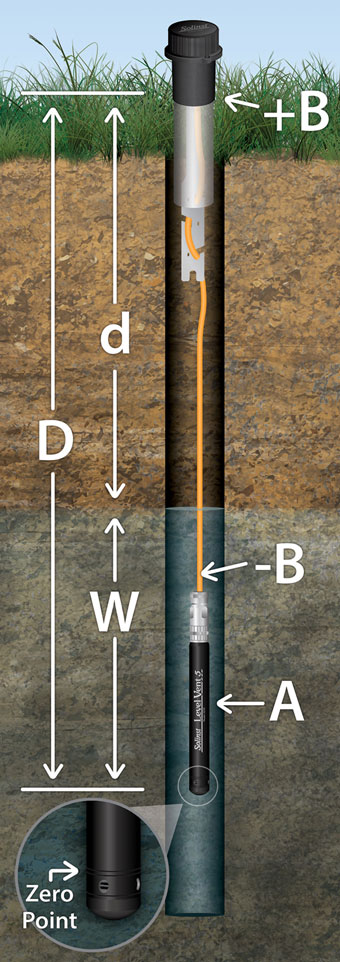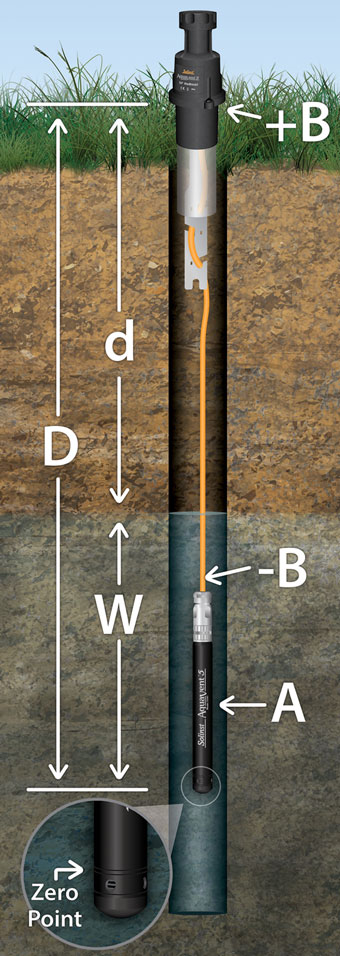# 1.2 Measurement Parameters

## 1.2.1 LevelFigure 1-3 Vented Logger Measurement Fundamentals

LevelVent and AquaVent detect both water (W) and barometric pressure (+B). However, a vent tube to surface allows barometric effects on the pressure sensor to be eliminated (-B). Therefore, the LevelVent and AquaVent provide readings of the actual pressure of just water above the pressure sensor zero point (A). This equation represents how the pressure sensors work:

A = (W + +B) + (-B)
Therefore, A=W (See Figure 1-2).

A     =     LevelVent/AquaVent readings
W    =    Water column height
+B   =    Barometric pressure detected by the pressor sensor
-B    =    Barometric pressure applied through the vented cable

A     =    D (depth of AquaVent Zero Point) - d (depth to water measurement)